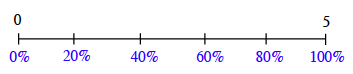### Home > CC1 > Chapter 3 > Lesson 3.1.2 > Problem3-32

3-32.

At Cassie’s Cashew Shoppe, a sign says, “Today only: $20\%$ off anything.” Maribel realizes that she has a coupon for $\frac { 1 } { 5 }$ off the price of anything in the shop. Which discount should she use, the $20\%$-off deal or the $\frac { 1 } { 5 }$-off coupon? Does it matter? Explain.

$20\%$ is the same as $\frac{20}{100}$. Can you use a percent ruler or number line to represent this?

Using the same ruler or number line to represent $\frac{1}{5}$ and $20\%$ might help you compare the portions.
Remember that one whole, or $100\%$, is the same as $\frac{5}{5}$.

Your number line might resemble the one below. Can you place $20\%$ and $\frac{1}{5}$When you plot the portions, what do you find? Remember to explain your answer!